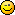## News:

*NEW* Current Version on STEAM16.793

Webchat:
Visit the chat

#### Kitty Hello

Code (glbasic) Select
`// my_drawangle - angle you can pass to ROTOSPRITE for correct orientation//// return:// 0 = bottom is at bottom// 1 = button is left// 2 = button is on top// 3 = button is rightFUNCTION iPadOrient%: BYREF my_drawangleLOCAL fx, fySTATIC last%, drawangle fx = GETJOYX(0) fy = GETJOYY(0) LOCAL limit = .75 IF fy < -limit THEN last = 0 IF fx >  limit THEN last = 1 IF fy >  limit THEN last = 2 IF fx < -limit THEN last = 3 LOCAL angle = last * 90 IF ABS(angle - drawangle)>180.0 IF angle < drawangle INC angle, 360.0 ELSE DEC angle, 360.0 ENDIF ENDIF my_drawangle = drawangle * 0.9 + angle*0.1; drawangle=my_drawangle RETURN lastENDFUNCTION`

#### Dark Schneider

#1
That is not exactly correct, the return values are wrong (the hurry).
Also it can be slighty modified to allow user to select the limit, the final function could be like:

Code (glbasic) Select
`// --------------------------------- //// my_drawangle - angle you can pass to ROTOSPRITE for correct orientation//// return:// 0 = bottom is at right// 1 = button is bottom// 2 = button is on left// 3 = button is topFUNCTION iPadOrient%: BYREF my_drawangle, limit#=0.75LOCAL fx, fySTATIC last%, drawangle   fx = GETJOYX(0)   fy = GETJOYY(0)   IF fy < -limit THEN last = 0   IF fx >  limit THEN last = 1   IF fy >  limit THEN last = 2   IF fx < -limit THEN last = 3   LOCAL angle = last * 90   IF ABS(angle - drawangle)>180.0      IF angle < drawangle         INC angle, 360.0      ELSE         DEC angle, 360.0      ENDIF   ENDIF   my_drawangle = drawangle * 0.9 + angle*0.1; drawangle=my_drawangle   RETURN lastENDFUNCTION`

Very good function